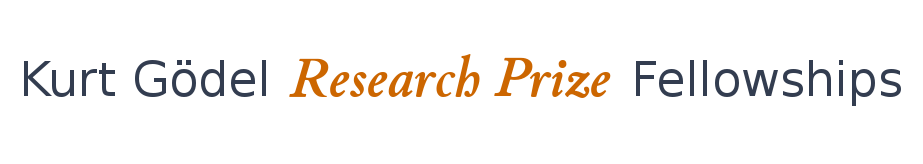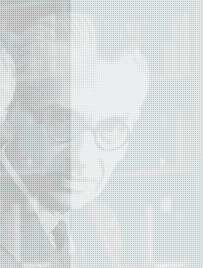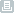HomeFinalists and WinnersPrevious FellowshipsTerms and ConditionsPrint this page

# Model Questions for Logical Foundations of AI

## INTUITION

One of the most effective expressions of intelligence is intuition. We do not have to deduce what we see. On the other hand, intuition makes us vulnerable to illusions. There is, however, a lack of an exact (i.e. mathematical) understanding of intuition. The following questions provide a basis for logical analysis:
1. Explain, why semantic methods ("seeing") are usually superior to syntactic ("deducing") methods in logic. Make the hidden deductions in semantic arguments explicit and classify the hidden decisions to obtain a formal theory of (at first mathematical) intuition.
2. Determine the closure operations, which generate suitable semantics from proof methods. What makes such semantics rich enough to be more natural and useful than the underlying proof methods?

## INFORMATION REDUCTION

Intelligence in the sense of cognitive ability does not so much depend on information collection but on suitable information deletion.The following questions are a starting point for a stable theory of information deletion w.r.t. decisions.
1. Develop a generative representation of proofs. (Whenever we think of a tree, it seems to us that we remember every single leaf, which is absurd. The picture of authentic leaves that is in our memory is replaced by a generative principle, which is as good. The same holds for mathematicians who think they have proofs in mind. A generative representation of proofs is therefore closer to the actual representation in our mind.)
2. Determine for various representations of proofs the minimal information (invariants) that allow one to reconstruct the proofs.

## CREATIVITY

In a formal sense, creativity is based on complicated transformations beyond mere derivability. (In the language of Mathematical Logic: these transformations are not conservative.) To automatize creativity, the following problems are a first starting point:
1. Develop logical mechanisms to derive general statements from examples. (This corresponds to the Babylonian origins of mathematics, where general statements were demonstrated for concrete numbers such that the generality was obvious.)
2. Develop a Proof Theory of Analogical Reasoning. (Analogical Reasoning is always a combination of generalization followed by instantiation. It is therefore always a theory of proof invariants.)

## UNBOUNDEDNESS and the INFINITE

Artificial Intelligence applications usually do not specify their limits in space and time. However, they need a concrete representation of the infinite.
1. Use Hilbert's epsilon notation as a first step to develop a finite representation of the infinite.
2. Specify conditions, where concrete objects (e.g. numerals) are generic (i.e. have the same meaning as variables) in order to reduce the infinite to the finite. (A straightforward example suggested by Gödel is the occurrence of an extremely large number in a very short proof.)

## COOPERATION

There is a lot of mainstream research in Artificial Intelligence concerning cooperation mainly based on the concept of agents. The following nonstandard problems deal with cooperative proofs and might turn out to be even more relevant for Mathematical Logic than for Artificial Intelligence.
1. Develop a theory of problem partition for a suitable field of mathematics. (The idea is that proofs are developed in parallel by a group of mathematicians such that the combination of the proofs solves the specified problem without the mathematicians being forced to fully understand the proofs of other members of the group.)
2. Develop a theory of formal crosschecks for proofs for a suitable field of mathematics, which render the correctness of proofs plausible. Classify the plausibility involved.

## LOCAL SYSTEMS OF LOGIC

Many problems in Artificial Intelligence are connected to the foundational problem of choosing the right logic, even more so when the logic of choice has to be changed during the execution of the application.
1. Develop a general logical framework that allows one to instantiate generic logics to usual logics and their proof systems according to the requirements of a specification.
2. Develop a meta-calculus to determine the adequacy of a formally represented logic for a formally represented problem.

## COMPLEXITY and SIMPLICITY

Complexity measures tend to focus on syntactic complexity only, which is machine oriented, and far from our natural understanding of complexity originating from structure. We therefore, suggest two alternative approaches:
1. Develop a general complexity theory based on the topological genus of associated problem graphs. (The topological genus measures the structural complexity. This idea has been applied by Richard Statman to Natural Deduction Derivations understood as graphs.)
2. Develop a theory of simplicity by defining proofs as simple when they cannot be generalized. (This measure does not rank complex proofs, but it separates simple proofs from complex ones).

## CONSCIOUSNESS

Consciousness may be considered as a supreme form of intelligence. Up until now all approaches to analyze it in a formal sense and to automatize it have failed. The following ambitious foundational projects might provide a first starting point for the development of a formal theory of consciousness:
1. Develop a theory of local consistency, i.e. only simple contradictions according to a suitable measure are excluded. (Here it is assumed that the main feature of consciousness is to provide a homogeneous environment and security for the immediate future.)
2. Develop a model theory for locally consistent theories. (Social perceptions of reality, ethics, religion etc. all depend on joined "consciousness-es".)